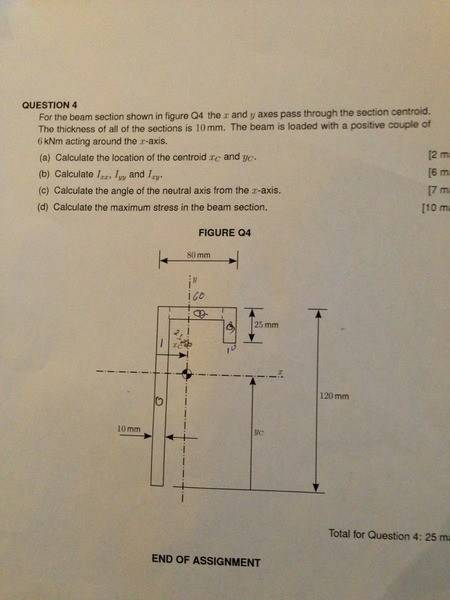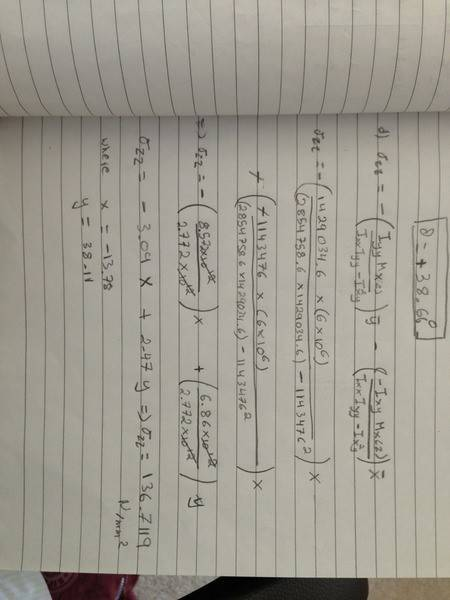# Calculate the angle of the neutral axis from the x-axis

• vtaela

## Homework Statement

Calculate the angle of the neutral axis from the x-axis when the beam is loaded with a positive couple of 6kNm acting around the X-axis

## Homework Equations

i have calculated:
Ixx: 2854758.6 mm^4
Iyy: 1429034.6 mm^4
Ixy: -1143476 mm^4

## The Attempt at a Solution

i know that to calculate the angle:

tanθ= - (IxxMy -IxyMx)/(IyyMx-IxyMy)

since i am confused with the statement "positive couple of 6kNm acting around the X-axis"

does that mean that Mx= 6KNm?

thank you

Ya got any pictures of this problem?This is the the question

Mx is a couple which is acting as a bending moment on the cross section.

Does that mean Mx = 6KNm?
That is correct.

(1) By the way, always leave a space between a numeric value and its following unit symbol. E.g., 6 kN, not 6kN. See the international standard for writing units (ISO 31-0).

(2) The unit symbol for kiloNewton is spelled kN, not KN. Capital K means kelvin.

(3) Two unit symbols multiplied together cannot be written together, and must be separated by, e.g., an asterisk or a space. E.g., kN*m or kN m, not kNm.

Last edited:
Thank u! I will have it in mind! In addition my ixy when i calculate it is positive and when i got the answer from solidworks i get a negative value! Which one is the correct?

vtaela: Although I have not tried it, it looks like Ixy perhaps should be positive. I do not know what SolidWorks is doing. Ensure your positive axes are pointing in the correct direction in SolidWorks.

Thank you very much

## Homework Equations

i have calculated:
Ixx: 2854758.6 mm^4
Iyy: 1429034.6 mm^4
Ixy: -1143476 mm^4

I got this except the Ixy was plus 1143476. Is this what you got when you did it again?

Yes i calculates a positive ixy and a positive 38.66 degree

When calculating the maximum stress did you use the unsymmetric bending equation? If you did what did you use as your x and y co-ordinates to input in that equation?

X = -13.78 and y+= 38.11 getting a maximum stress of 136.7119

How did you get them? Did you draw it out or is there a way to calculate them?

Actually think i understand it.

X = Xc - bredth of rectangle 1
Y = height of rectangle 1 - Yc

That how you got them?

Yes that's right

Are you sure about your final stress value? I get 151.9855 N/mm^2 every time.

I've tried using hand calculations as well as using excel to double check the values and get that everytime.

Take a picture of what you have done to compare the solution! I will reply tmr since i am not home now

Same procedure except i used a positive Mx

Any idea how to do question 1 of the coursework?:p

Can you stick a pic of your solution up please. I completely missed the positive couple part in the question so can see where the positive mx comes from but now i now get -117.879 - 34.1061 = -151.99! Absolute nightmare haha.

Haha no idea, think everyone is stumped with that one.Cheers, i think you have your x and the y mixed up at the end. You have -3.09x + 2.475y when it should be -3.09y + 2.475x according to the equation?

Yes you are right thank you for that

No problem